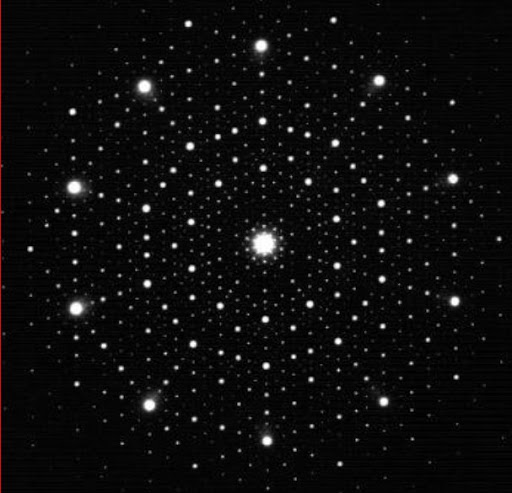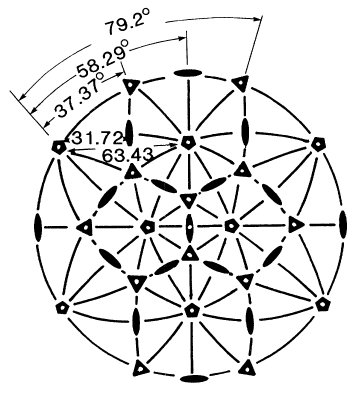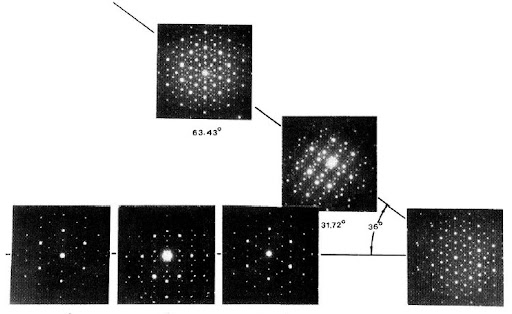### The mathematics in the 2011 Nobel Prize in Chemistry

The Nobel Prize in Chemistry 2011 is assigned to Daniel Shechtman
for the discovery of quasicrystalsThe paper of the discover, written with Blech, Gratias and Cahn, starting with the following worlds:
We report herein the existence of a metallic solid which diffracts electrons like a single crystal but has point group symmetry $m \bar{35}$ (icosahedral) which is inconsistent with lattice translations.(2)The lattice translations are, indeed, most important tools in order to classify crystals. Indeed in 1992 the definition of crystals given by the International Union of Crystallography was:
A crystal is a substance in which the constituent atoms, molecules, or ions are packed in a regularly ordered, repeating three-dimensional pattern.
So the discover of Shechtman and collegues was very important: they introduce a new class of crystals, named quasicrystals by Levine and Steinhardt some weeks later(3), and a new way to view crystals.
In particular Shechtman, studying Al with 10–14% Mn, and collegues observed that
The symmetries of the crystals dictate that several icosahedra in a unit cell havedifferent orientations and allow them to be distorted (...)(2)
And when they observe crystal using lattice translations:
crystals cannot and do not exhibit the icosahedral point group symmetry.(2)
They also oserve that the formation of the icosahedral phase is a transition phase of the first order, because the two phases (the other is translational) coexist for a while during translation(2).But quasicrystals have also great interest in mathematics: for example De Bruijn showed(1) that Penrose tilings are particular bi-dimensional quasicrystals. And it's interesting to notice that Penrose tilings are related to golden ratio, that defines the coordinate system of an icosahedron.
Indeed we can define the coordinate system of the solid in the following way(4): $(\pm u, \pm v, 0); \; (0, \pm u, \pm v); \; (\pm v, 0, \pm u)$ where $\frac{v}{u} = \tau - 1$ with $\tau$ golden ratio.
So the elements of the icosahedral point groups are the symmetry transformations that permutate icosahedral vertices. With this definition, we can distinguish between two different icosahedral gruop, $235 [I]$ that contains all rotations which leave the icosahedron invariant, and $2/M \bar{35} [I_h]$, the group of Al with 10–14% Mn, that contains 120 elements.
This group is the direct product of the icosahedral group $235 [I]$ and the group consisting of the identity and spatial inversion.(4)
The four generators of the group can be represented with the following matrices: $D [2 (12)] = \begin{pmatrix} -1 & 0 & 0 \\ 0 & -1 & 0 \\ 0 & 0 & 1 \end{pmatrix}$ $D [3 (143)] = \begin{pmatrix} 0 & 0 & 1 \\ 1 & 0 & 0 \\ 0 & 1 & 0 \end{pmatrix}$ $D [5 (1-12)] = \begin{pmatrix} 0.5 & -0.5 \tau & \frac{0.5}{\tau} \\ 0.5 \tau & \frac{0.5}{\tau} & -0.5 \\ \frac{0.5}{\tau} & 0.5 & 0.5 \tau \end{pmatrix}$ $D [\bar{1}] = \begin{pmatrix} -1 & 0 & 0 \\ 0 & -1 & 0 \\ 0 & 0 & -1 \end{pmatrix}$
Disorderly quasicrystals
Icosahedral group
Easter Is a Quasicrystal
Nobel Prize in Chemistry: Further readings
(1) de Bruijn, N. (1981). "Algebraic theory of Penrose's non-periodic tilings of the plane". Nederl. Akad. Wetensch. Proc A84: 39.
(2) Shechtman, D., Blech, I., Gratias, D., & Cahn, J. (1984). Metallic Phase with Long-Range Orientational Order and No Translational Symmetry Physical Review Letters, 53 (20), 1951-1953 DOI: 10.1103/PhysRevLett.53.1951
(3) D. Levine, R. Steinhardt (1984) ―Quasicrystals: a new class of ordered structures‖, Physical Review Letters 53(26), pp 2477-2480.
(4) Litvin, D. (1991). The icosahedral point groups Acta Crystallographica Section A Foundations of Crystallography, 47 (2), 70-73 DOI: 10.1107/S0108767390010054